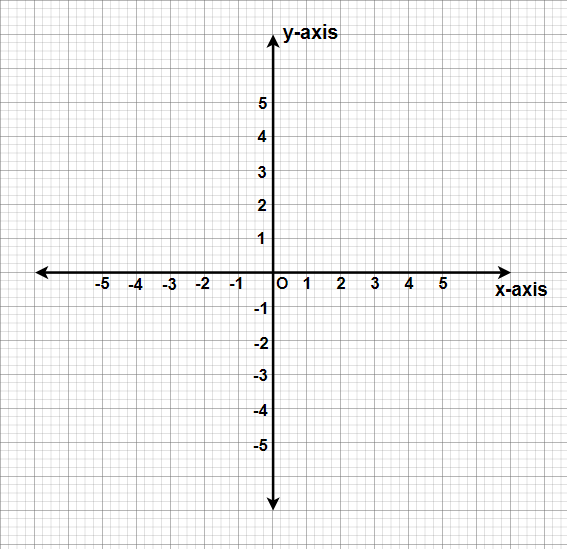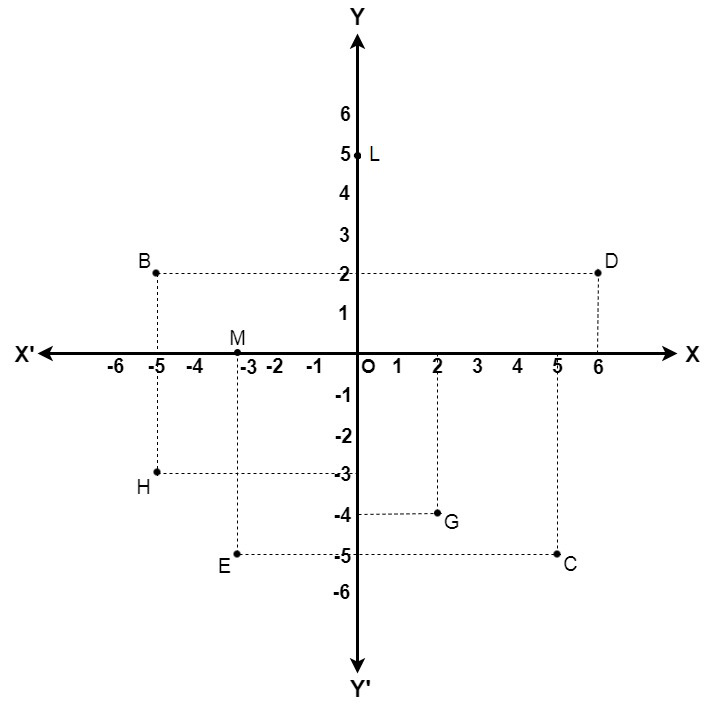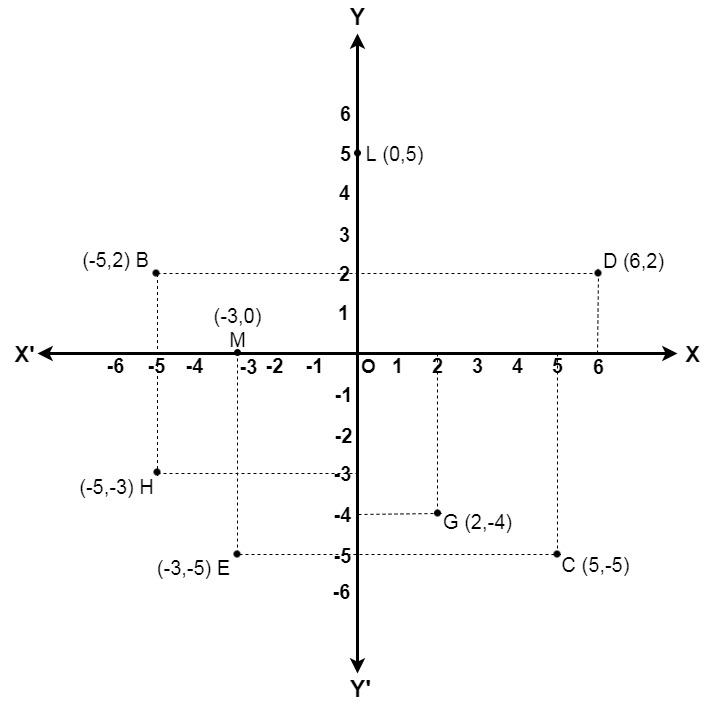# Class 9 NCERT Solutions – Chapter 3 Coordinate Geometry – Exercise 3.2

• Last Updated : 04 Jan, 2021

### (i) What is the name of the horizontal and the vertical lines drawn to determine the position of any point in the Cartesian plane?(ii) What is the name of each part of the plane formed by these two lines?(iii) Write the name of the point where these two lines intersect.

Solution:

(i) Name of the horizontal and vertical lines are:

Attention reader! All those who say programming isn't for kids, just haven't met the right mentors yet. Join the  Demo Class for First Step to Coding Coursespecifically designed for students of class 8 to 12.

The students will get to learn more about the world of programming in these free classes which will definitely help them in making a wise career choice in the future.

• The horizontal line drawn on the Cartesian plane is known as x-axis.
• The vertical line drawn on the Cartesian plane is known as y-axis.(ii) The name of each part of the plane formed by the two lines x-axis and y-axis is called as a quadrant (1/4th part).

(iii) Name of the point where there two lines intersect is called the origin(O).

### Question 2: See the given figure, and write the following:(i) The coordinates of B.(ii) The coordinates of C.(iii) The point identified by the coordinates (–3, –5).(iv) The point identified by the coordinates (2, – 4).(v) The abscissa of the point D.(vi) The ordinate of the point H.(vii) The coordinates of the point L.(viii) The coordinates of the point M.Solution:

(i) The coordinates of point B is the distance of point B from x-axis and y-axis that is −5 and 2 respectively.
Therefore, the coordinates of point B are (−5, 2).

(ii)  The coordinates of point C is the distance of point C from x-axis and y-axis that is  5 and −5 respectively.
Therefore, the coordinates of point C are (5, −5).

(iii) The point whose x-coordinate and y-coordinate are −3 and −5 respectively is point E.

(iv) The point whose x-coordinate and y-coordinate are 2 and −4 respectively is point G.

(v) The x-coordinate of point D is 6. Therefore, the abscissa of point D is 6.

(vi) The y-coordinate of point H is −3. Therefore, the ordinate of point H is −3.

(vii) The coordinates of point L is the distance of point L from x-axis and y-axis that is 0 and 5 respectively.
Therefore, the coordinates of point L are (0, 5).

(viii) The coordinates of point M is the distance of point M from x-axis and y-axis that is −3 and 0 respectively. Therefore, the coordinates of point M is (−3, 0).My Personal Notes arrow_drop_up